## Recommended Posts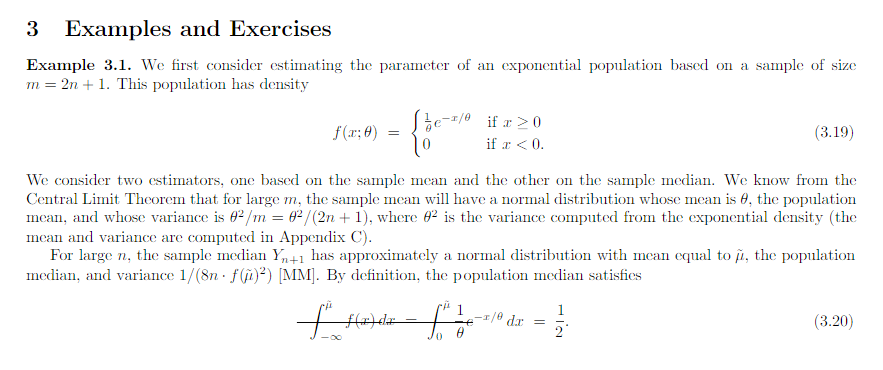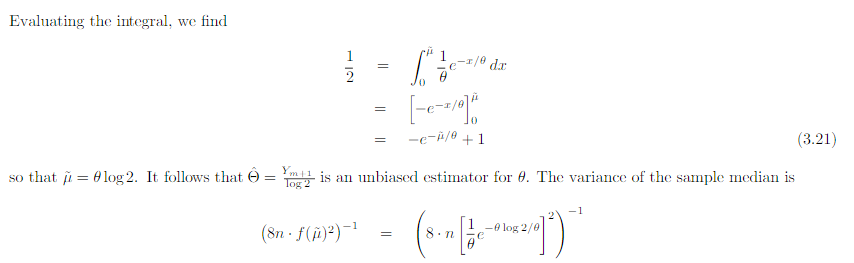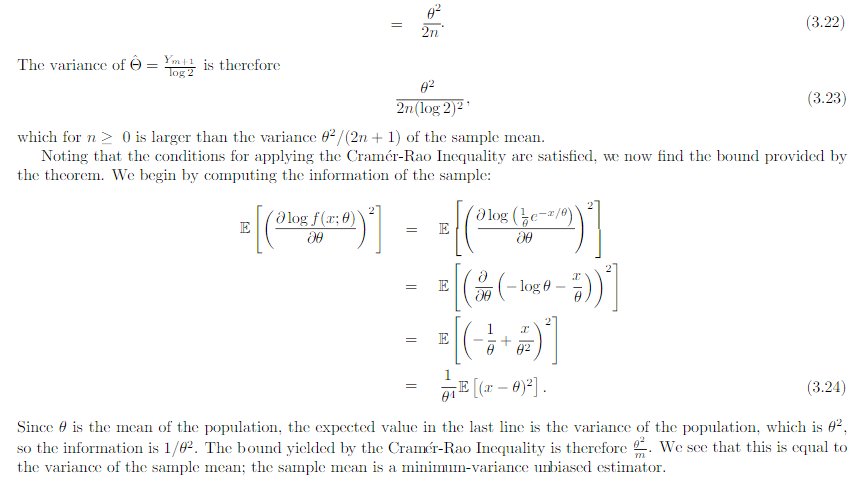In the example above they use the cramer rao minimum variance estimation. I have one problem. For the mean they use the pdf of exponential. However the mean of exponential follows gamma. And the median distribution can be shown to be normal distributed in general. Why do they use the exponential pdf in Cramer Rao when the mean is gamma distributed? And how can they compare the median and the mean with Cramer Rao when they follow different distributions. I have a derivation in my book of Cramer Rao that starts with mle and uses the pdf until it shows that the covariance between the estimator and the fisher information of the pdf is bounded. But is it not so that you must use the same pdf if you were to compare estimators. This last problem is what I need most clarified. How is it so that Cramer Rao be used if it compares estimators that follow different distributions? In the example above the estimators follow different distributions Thanks in advance! Below I have added how they derive Cramer Rao bound in my book with a comment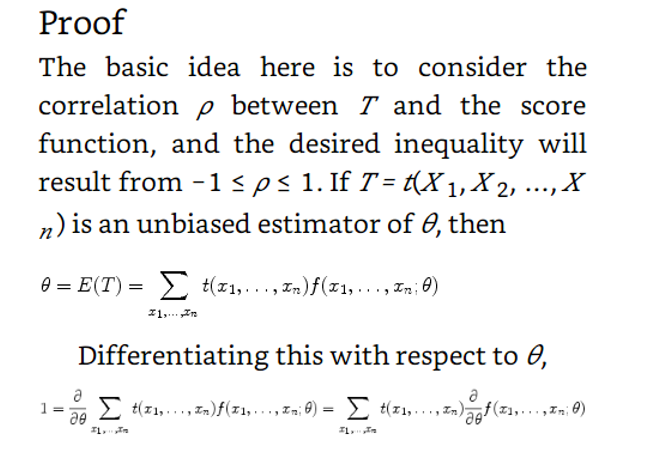Above they use the expected value of T where T is the estimator for example mean or median as in the example in the beginning of the question. But since they find the expected value

of T must not they then use the pdf that corresponds to T? Which in the example above would be gamma and normal respectively. How can then Cramer Rao bound compare anything

if it looks at the bound for different pdfs?

Here is the rest of the proof just in case

##### Share on other sites

What is the function $$f$$ which has only one argument but has the same name as the density function $$f$$ that has two arguments?

##### Share on other sites

I will add the start of the theory that obtains the fisher information from the maximum likelihood function

My notes from this theory is that they talk about the maximum likelihood estimator and that they introduce a sample which should be T in the theory in the first post. My question is still the same:

Above they use the expected value of T where T is the estimator for example mean or median as in the example in the beginning of the question. But since they find the expected value

of T must not they then use the pdf that corresponds to T? Which in the example above would be gamma and normal respectively. How can then Cramer Rao bound compare anything

Just for clarification. The example in the beginning of the first post is not from the rest of the theory. The theory after the example in the first post comes just after this theory added in this post in the chapter of the theory is taken from.

##### Share on other sites
15 hours ago, Tor Fredrik said:

Above they use the expected value of T where T is the estimator for example mean or median as in the example in the beginning of the question. But since they find the expected value

of T must not they then use the pdf that corresponds to T? Which in the example above would be gamma and normal respectively.

I do not understand this part of your question. The point is that $$t$$ can be any estimator for $$\theta,$$ providing the expected value of $$t$$ is actually equal to $$\theta.$$ You are not assuming any particular pdf for $$T,$$ except that which is given by the pdf $$f$$ for the individual outcomes $$x_1,\ldots,x_n.$$

##### Share on other sitesSo how do you interpretate this. For example for a normal distribution. I have an assignment about this in my textbook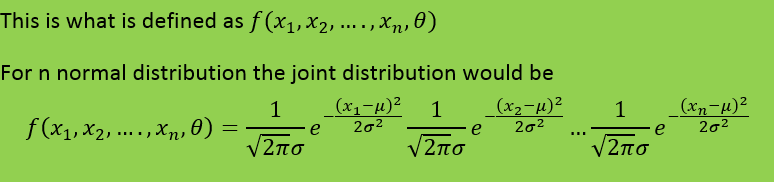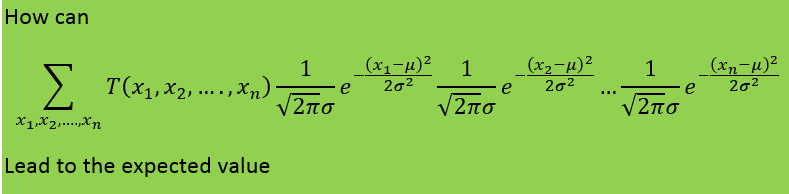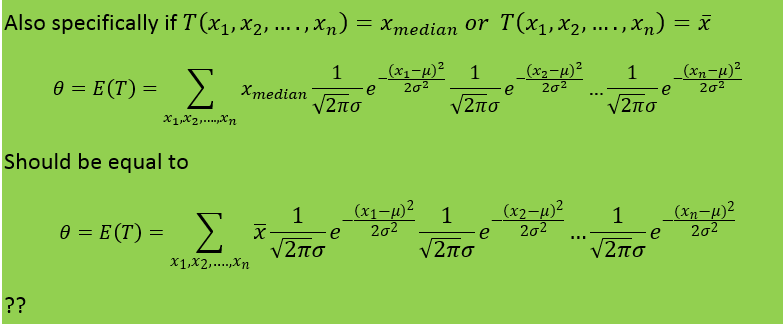It would be easier if someone could show me directly how this is valid

Edited by Tor Fredrik

##### Share on other sites

Generally you could use integral notation, and in the continuous case, like for the normal distribution, you should do so. Integral notation covers both cases.

I don't remember how to work out the expected value of the median estimator for $$\theta.$$

For the average estimator it should be fairly straightforward. With $$T=\bar{x}=\frac{1}{n}\sum_{i=1}^n x_i$$ we get

$E(T) = \int_{x_1,\ldots,x_n} \frac{1}{n}(\sum_{i=1}^n x_i) \prod_{i=1}^n \frac{1}{\sqrt{2\pi}\sigma} e^{\frac{(x_i-\mu)^2}{2\sigma^2}}dx_1\cdots dx_n.$

Actually after simplification it is just the sum of the expected values of each $$x_i$$ divided by $$n$$. Since $$E(x_i)=\mu,$$ we get $$E(T)=\mu$$. I hesitate to work out the details now, because I am not at home and only have my little notebook available.

Edited by taeto

## Create an account

Register a new account